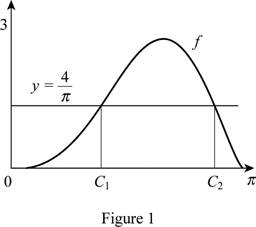# The average value of the function f ( x ) = 2 sin x − sin 2 x### Single Variable Calculus: Concepts...

4th Edition
James Stewart
Publisher: Cengage Learning
ISBN: 9781337687805### Single Variable Calculus: Concepts...

4th Edition
James Stewart
Publisher: Cengage Learning
ISBN: 9781337687805

#### Solutions

Chapter 6.5, Problem 9E

(a)

To determine

## To find: The average value of the function f(x)=2sinx−sin2x

Expert Solution

The average value of the function is 4π.

### Explanation of Solution

Given information:

The function is f(x)=2sinxsin2x,[0,π].

Calculation:

Show the function:

f(x)=2sinxsin2x (1)

The expression to find the average value of the function (favg) is shown below:

fave=1baabf(x)dx (2)

Here, the upper limit is b, the lower limit is a, and the function is f(x).

Substitute 0 for a, π  for b and 2sinxsin2x for f(x) in Equation (2).

fave=1π00π(2sinxsin2x)dx=1π0π(2sinxsin2x)dx (3)

Integrate Equation (3) with respect to x and apply the limits.

fave=1π[2cosx+12cos2x]0π=1π[2+12(2+12)]=4π

Thus, the average value of the function is 4π.

(b)

To determine

### To find: The value of count c.

Expert Solution

The value of c is 1.238 or 2.808.

### Explanation of Solution

Given information:

The function is f(x)=2sinxsin2x,[0,π].

Calculation:

Find the value of c using the formula:

f(c)=fave (4)

Substitute 4π for fave in Equation (4).

f(c)=4π

Substitute c for x in Equation (1)

f(c)=2sincsin2c (5)

Substitute 4π for f(c) in Equation (5).

2sincsin2c=4π (6)

Solve Equation (6) using excel solver.

2sincsin2c=4πc=c11.238c=c22.808

Thus, the value of c is 1.238 or 2.808.

(c)

To determine

Expert Solution

### Explanation of Solution

Substitute 1.238 for x in Equation (1)

y=2sin1.238sin1.238=1.8900.945=0.944

Substitute 2.808 for x in Equation (1)

y=2sin2.808sin2.808=0.6540.327=0.327

Draw the graph for the curve function y=2sinxsin2x and mark the coordinates (1.238, 0.944) (2.808, 0.327) as shown in Figure 1.### Have a homework question?

Subscribe to bartleby learn! Ask subject matter experts 30 homework questions each month. Plus, you’ll have access to millions of step-by-step textbook answers!